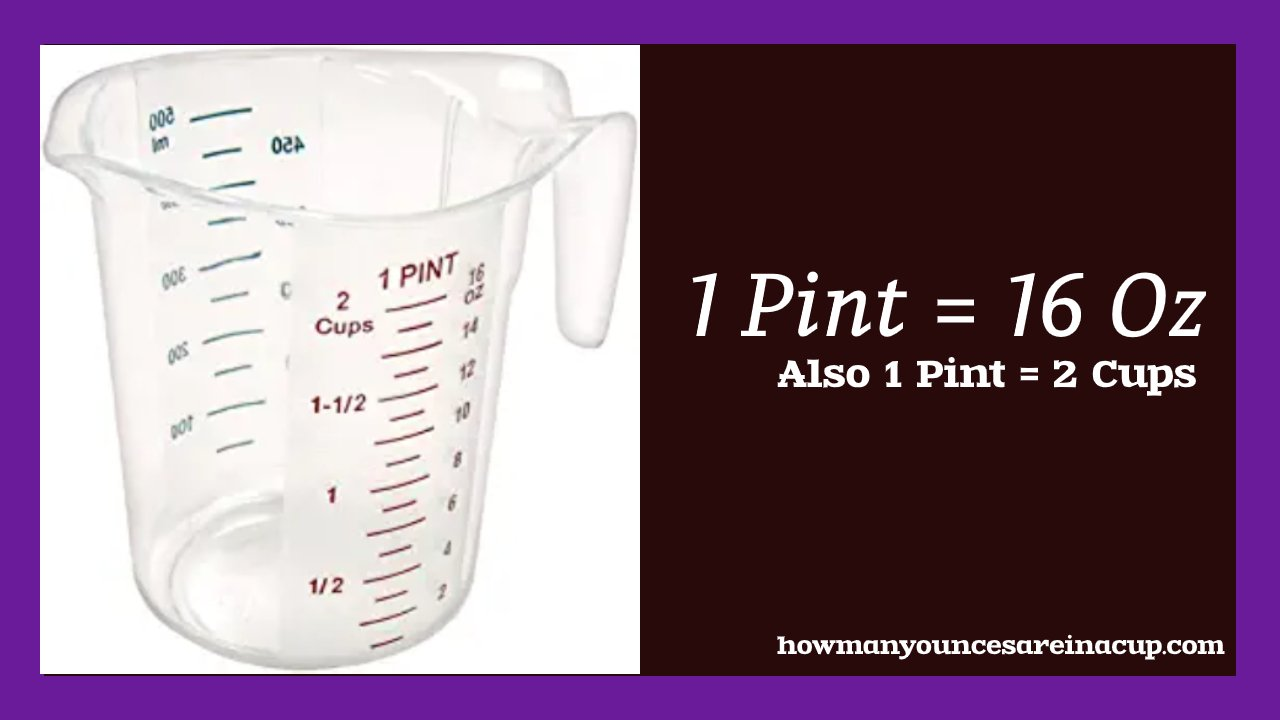# How Many Ounces are in a Pint?

1 pint = 16 ounces. It is easy to convert a pint to ounces in a converter. You just have to give enough information to get more possible answers. Depending upon the substance, the standard measurement of a pint varies. When using the US and imperial standards, you find results may vary. This is one of the ways used to measure the weight of the substance in our day to day life. As you see, pints of milk and beer are the most considered units of volume or density of the substance.## Conversion

It is good to know how the single pint is converted to a variety of ounces. Want to convert a pint into either Imperial or US sized pint? If you try to convert from a dry pint to ounces or if the substance is a liquid pint, you can find a significant difference at the end of conversion. These facts are ensured to meet the following standards as listed below

How many ounces in dry pint(US)?

As you see, the first conversion is related to the dry pint. The total volume found in this dry pint is 18.62 ounces.

How many ounces in a Liquid Pint(US)?

This slightly differs from the above conversion. Do you know that a liquid pint (US) is equal to 16 fluid ounces.

The only difference between the above two conversions is because of the uneven number of ounces that are present in the liquid and a dry pint.

How many ounces in an Imperial pint?

The UK is the only country that uses this imperial pint measurement. It is 20% larger than the unit of measurement in the US. This finally makes the conversion of an imperial pint is equal to 20 ounces.

Check how many ounces in a Pint of Ice Cream?

It is found that 16 ounces are found in a pint of ice cream.

How many ounces in a Pint of Blueberries?

In general, blueberries are often measured in a dry form. Its dry nature accounts for approximately 2 cups. That in turn, sums up to the measurement of 12 ounces.

Note: The units of measurement vary and depend upon the size and weight of the fruits. Fruit can be anything like a small compact blueberry or larger strawberry will modify the number of ounces where a pint will weigh.

How many ounces in a pint of strawberries?

Strawberry is a larger berry than requires more fruit to calculate a pint in it. You may think it is difficult to convert these items to ounces. It just requires a little knowledge to measure the weight of the substances.

The ounce differs when the berries are either whole or sliced pieces. Whole strawberries equal to a pint of 3.25 cups of fruit whereas the sliced berries equal to a pint of 2 cups and a pint of pureed strawberries is equal to 1.5 cups of berries.

On the other side, One cup of strawberries equals 7.1 ounces in total.

Now the weight of a pint of whole strawberries = 23.075 ounces

Pint of sliced strawberries = 14.2 Ounces.

Pint of Pureed strawberries = 10.65 ounces.

Finally, all these are mentioned above to let you know that 1 cup merely equals 7.1 Ounces.